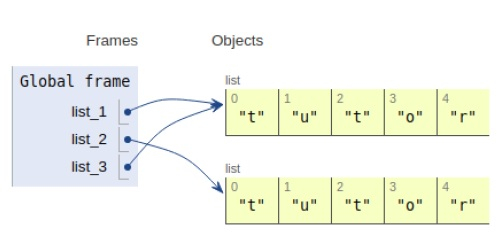# Python Program for Common Divisors of Two Numbers

PythonServer Side ProgrammingProgramming

Problem statement − We are given two integers, we need to display the common divisors of two numbers

Here we are computing the minimum of the two numbers we take as input. A loop to calculate the divisors by computed by dividing each value from 1 to the minimum computed

Each time the condition is evaluated to be true counter is incremented by one.

Now let’s observe the concept in the implementation below−

## Example

Live Demo

a = 5
b = 45
count = 0
for i in range(1, min(a, b)+1):
if a%i==0 and b%i==0:
count+=1
print(count)

## Output

2All the variables are declared in the local scope and their references are seen in the figure above.

## Conclusion

In this article, we have learned about the python program to find the common divisors of two numbers.